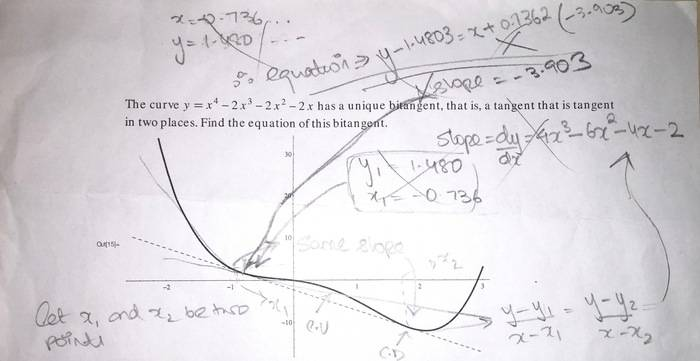# Finding the equation of a bitangent line to a curve

• I
• Kyrie

#### Kyrie

The curve $y=x^4-2x^3-2x^2-2x$ has a bitangent. I need to find the equation of this line.

First, I started off by computing the slope. Since it touches two points on the curve, their slopes should be the same.
So, I have the equation $4x^3_1-6x^2_1-4x_1-2=4x^3_2-6x^2_2-4x_2-2$
I got up to the point where I have $x^2_1+x_1x_2+x^2_2 = 3/2(x_1+x_2) + 1$
I seem to be stuck here. Any help?

Last edited:
Oh, guess I have no idea how to format the equation. My bad!

The curve $y=x^4-2x^3-2x^2-2x$ has a bitangent. I need to find the equation of this line.

First, I started off by computing the slope. Since it touches two points on the curve, their slopes should be the same.
So, I have the equation $4x^3_1-6x^2_1-4x_1-2=4x^3_2-6x^2_2-4x_2-2$
I got up to the point where I have $x^2_1+x_1x_2+x^2_2 = 3/2(x_1+x_2) + 1$
I seem to be stuck here. Any help?
Or ##2x_1^2 + 2x_1x_2 + 2x_2^2 - 3x_1 - 3x_2 - 2 = 0##
You could solve for, say, ##x_1## in terms of ##x_2##, using the Quadratic Formula. You'll need another equation to be able to determine ##x_1## uniquely. The points of tangency are on the graph of your original fourth-degree function, so I think you can get your second equation using that idea.

Or ##2x_1^2 + 2x_1x_2 + 2x_2^2 - 3x_1 - 3x_2 - 2 = 0##
You could solve for, say, ##x_1## in terms of ##x_2##, using the Quadratic Formula. You'll need another equation to be able to determine ##x_1## uniquely. The points of tangency are on the graph of your original fourth-degree function, so I think you can get your second equation using that idea.
Alright, solving for ##x_1##, I got ##x_1=\frac{-2x_2 + 3 \pm \sqrt{4x_2^4 + 4x_2^2 + 24x_2 - 7}}{4}##
I'm not sure if I have the points of tangency. The only equation relating ##x_1## and ##x_2## are the fact that they have the same slope.
I'll attach a picture of the question if that helps. P.S. sorry for the scribbles :(Let's say that the left tangent point goes through ##(x_1, y_1)##, with ##x_1## slightly to the right of -1. You can write the equation of the tangent line through ##(x_1, y_1)##, with everything in terms of ##x_1##. By that, I mean ##y_1 = x_1^4 - 2x_1^3 - 2x_1^2 - 2x_1##, and the slope is ##m = 4x_1^3 - 6x_1^2 - 4x_1 - 2##.
The equation of the tangent line is ##y - y_1 = m(x - x_1)## or ##y = y_1 + m(x - x_1)##. Find the point on this line for which the y-value on the line is equal to the y-value on the curve.

The two points we're looking for have to satisfy two criteria: 1) the slope has to be the same at both (the equation you found), and 2) both points have to lie on the quartic curve. With that second equation I think you will be able to find the coordinates of the two points of tangency.

Last edited: